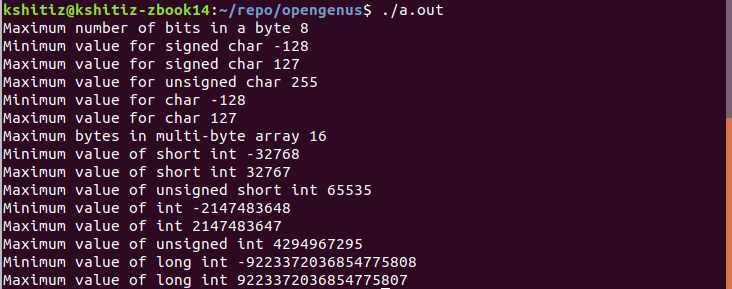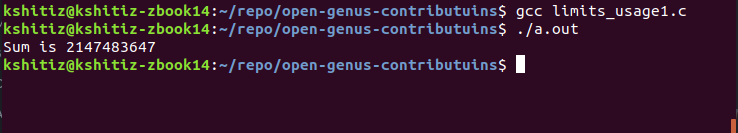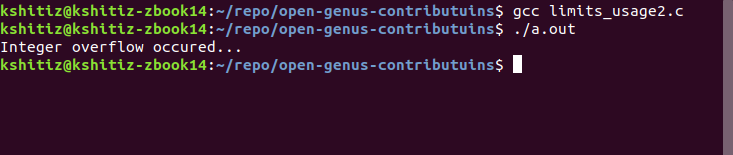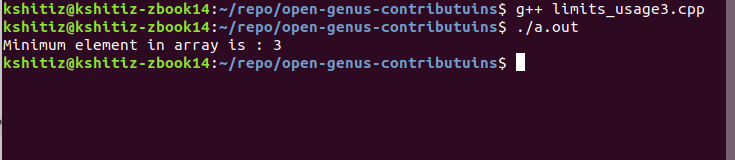×

Search anything:

# Working with limits.h in C

#### Software Engineering CGet this book -> Problems on Array: For Interviews and Competitive Programming

Reading time: 20 minutes | Coding time: 10 minutes

Limits.h header file in C is used to determine the set limits of variable data stypes. Macros defined in limits.h are used to find the limit of the values of various data types. The defined limits specify that any variable can not store any value beyond these limits.

To include this header file, use the following code segment:

``````#include <limits.h>
``````

## Library Macros

The defined values are implementation specific and are defined using the #define directive. Following are the macros defined in the header file.

Macro Value Description
CHAR_BIT 8 Maximum number of bits in a byte
SCHAR_MIN -128 Minimum value for signed char
SCHAR_MAX +127 Maximum value for signed char
UCHAR_MAX 225 Maximum value for unsigned char
CHAR_MIN -128 Minimum value for char type
CHAR_MAX +127 Maximum value for char type
MB_LEN_MAX 16 Maximum bytes in multi-byte array
SHRT_MIN -32768 Minimum value of short int
SHRT_MAX +32767 Maximum value of short int
USHRT_MAX 65535 Maximum value of unsigned short int
INT_MIN -2147483648 Minimum value of int
INT_MAX +2147483647 Maximum value of int
UINT_MAX 4294967295 Maximum value of unsigned int
LONG_MIN -9223372036854775808 Minimum value of long int
LONG_MAX +9223372036854775807 Maximum value of long int
ULONG_MAX 18446744073709551615 Maximum value of unsigned long int

Note: The above values are according to the GCC compilers and must be used in UPPER CASES.

If we want to find the upper limit of the value that can be stored in INT (integer data type), we need to use the INT_MAX macro.

After including the header file, it is accessed by:

``````int max = INT_MAX;
printf("Maximum value of int %d\n", INT_MAX);
``````

## Example code

In this example C code, we are printing the values of all macros defined in the limits.h header file.

``````#include <stdio.h>
#include <limits.h>

int main()
{

printf("Maximum bits in a byte %d\n", CHAR_BIT);

printf("Minimum value for signed char %d\n", SCHAR_MIN);
printf("Maximum value for signed char %d\n", SCHAR_MAX);
printf("Maximum value for unsigned char %d\n", UCHAR_MAX);

printf("Minimum value for char %d\n", CHAR_MIN);
printf("Maximum value for char %d\n", CHAR_MAX);

printf("Maximum bytes in multi-byte array %d\n", MB_LEN_MAX);

printf("Minimum value of short int %d\n", SHRT_MIN);
printf("Maximum value of short int %d\n", SHRT_MAX);
printf("Maximum value of unsigned short int %d\n", USHRT_MAX);

printf("Minimum value of int %d\n", INT_MIN);
printf("Maximum value of int %d\n", INT_MAX);
printf("Maximum value of unsigned int %ld\n", UINT_MAX);

printf("Minimum value of long int %ld\n", LONG_MIN);
printf("Maximum value of long int %ld\n", LONG_MAX);

return(0);
}
``````

After compiling and running the output is :## Using limits.h in code

In this example, we implemented a simple sum function which returns the sum of two values if there is no overflow. If there is overflow, then it is return INT_MIN.

Scenario 1 (sum within limit)

``````#include <stdio.h>
#include <limits.h>

int sum (int num1, int num2)
// Sum function returning sum if no overflow is present...
{
if (num1 > INT_MAX - num2)
return INT_MIN;

else
return num1 + num2;
}

int main()
{

int num1 = 2147483627;
int num2 = 20;
// Two numbers with sum in limits of integer

int result = sum(num1, num2);

if (result == INT_MIN)
{
printf("Integer overflow occured...");
}
else
{
printf("Sum is %d", result);
}
return 0;
}
``````

After compiling and running the output is :Scenario 2 (sum exceeding limit)

``````#include <stdio.h>
#include <limits.h>

int sum (int num1, int num2)
// Sum function returning sum if no overflow is present...
{
if (num1 > INT_MAX - num2)
return INT_MIN;

else
return num1 + num2;
}

int main()
{

int num1 = 2147483627;
int num2 = 2000;
// Two numbers with sum more than limits of integer

int result = sum(num1, num2);

if (result == INT_MIN)
{
printf("Integer overflow occured...");
}
else
{
printf("Sum is %d", result);
}
return 0;
}
``````

After compiling and running the output is :## Minimum element in an array

In this example, we will calculate the minimum element in an array.

``````#include <iostream>
#include <limits.h>

using namespace std;

void minimum(int array[], int size)
{
int minimm = INT_MAX;
for (int i=0;i<size;i++)
{
minimm=min(minimm, array[i]);
}
cout<<"Minimum element in array is : "<<minimm<<endl;
}

int main()
{
int array[] = { 345, 7645, 234345, 976, 3 };

int size = sizeof(array)/sizeof(array);

minimum(array,size);

return 0;
}
``````

After compiling and running the output is :With this, you have a working knowledge of using limits.h and this is an useful knowledge as you can implemented ideas keeping in mind the limits of data types in C and work around to avoid any runtime errors or unexpected results.

Enjoy.#### Kshitiz Saini

(DevOps + Linux) Enthusiast | Bachelor of Technology Computer Science with specialization in DevOps (2018-2022) | Learner | Intern at OpenGenus

Working with limits.h in C